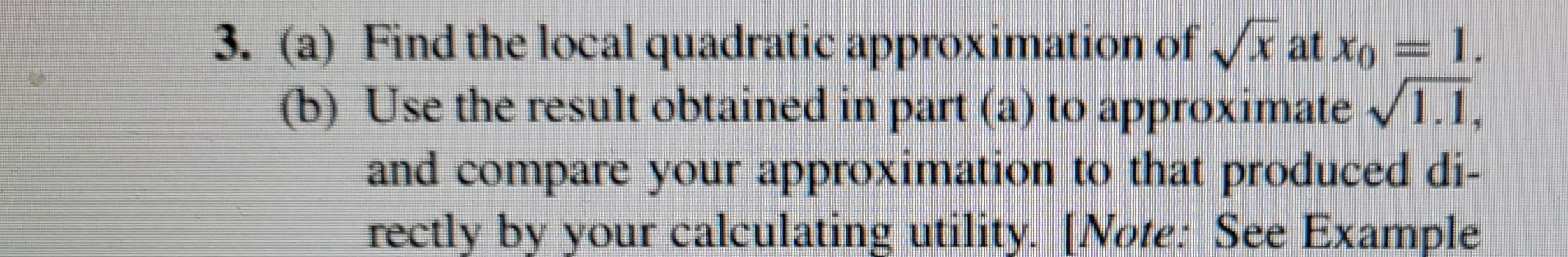### ¿Todavía tienes preguntas de matemáticas?

Pregunte a nuestros tutores expertos
Algebra
Pregunta3. (a) Find the local quadratic approximation of $$\sqrt { x }$$ at $$x _ { 0 } = 1$$ . (b) Use the result obtained in part (a) to approximate $$\sqrt { 1.1 }$$ , and compare your approximation to that produced di- rectly by your calculating utility. [Note: See Example

$$f(x)= \sqrt{x}\\f'(x)= \frac{1}{2}x^{- \frac{1}{2} } \\f''(x)= - \frac{1}{4}x^{- \frac{3}{2} } \\\Rightarrow f(x_{0})= 1,f'(x_{0})= \frac{1}{2}, f''(x_{0})= - \frac{1}{4} \\\Rightarrow f(x)\approx 1+ \frac{1}{2}(x- 1)- \frac{1}{8}(x- 1)^{2}$$